The 2D flowmap node calculates a flow map of water flows, similar to the Flow simulation node.

• The Flow simulation node produces a map of water flows, which translates, when converted to a mask, to a black and white image.
• The 2D flowmap node produces a vector map. The direction of each vector corresponds to the direction of the water flow. The norm of each vector corresponds to the water flow.

The vectors are stored in the same way as for a normal map:

• The red channel stores the X component of the vector: from 0 (toward the left) to 0.5 (in the center, therefore a zero component) up to 1 (to the right).
• The green channel stores the Y component of the vector.
• The blue channel stores the Z component of the vector, which is always equal to 0 since it is a 2-dimensional map and translates to a value of 0.5 stored in the blue channel.

To add a 2D flowmap node, right-click in the Graph Editor and select Create Node > Simulation > 2D flowmap or press the keyboard shortcut N2F (Node 2D Flowmap).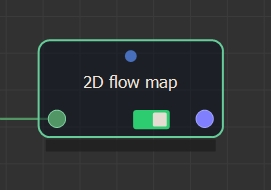Double click on the node to open its parameters: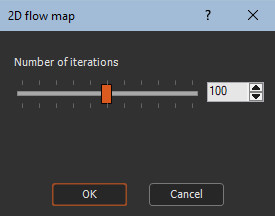Editing a 2D flowmap node

Use the slider the change the number of iterations.

Examples

Below is an example of calculated 2D flowmaps: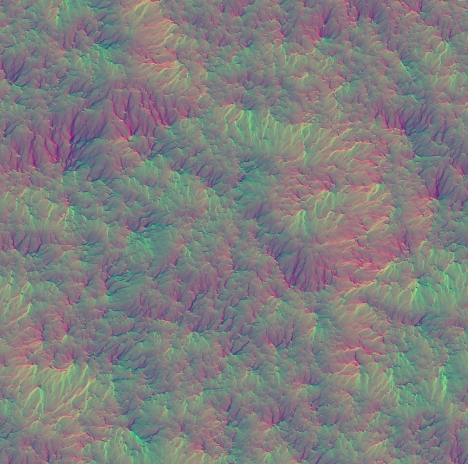You can export this vector map using the Export vector map or Multi file export vector map nodes.

You can also convert this vector map to a color map using the Vector map to color map node, which then allows you to apply the color map in the terrain.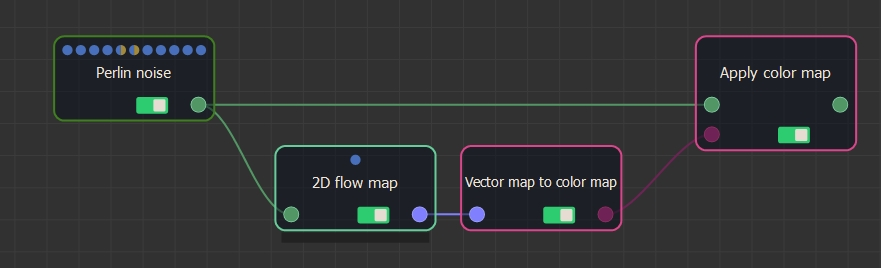Below is an example of a 2D flowmap converted to color maps and applied to the input terrain.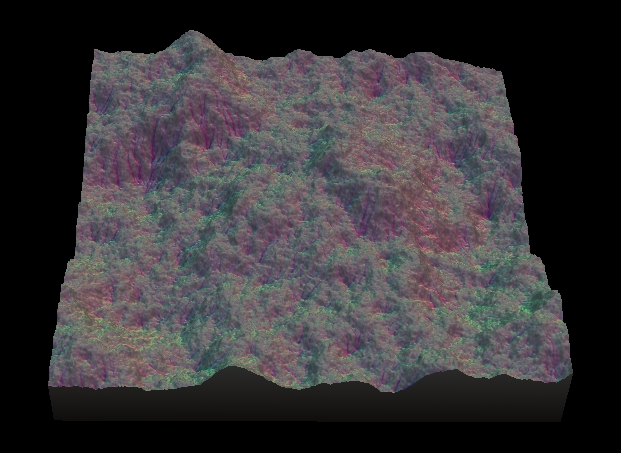Parameters

Parameter Description
Number of iterations Sets the number of iterations of the simulation.